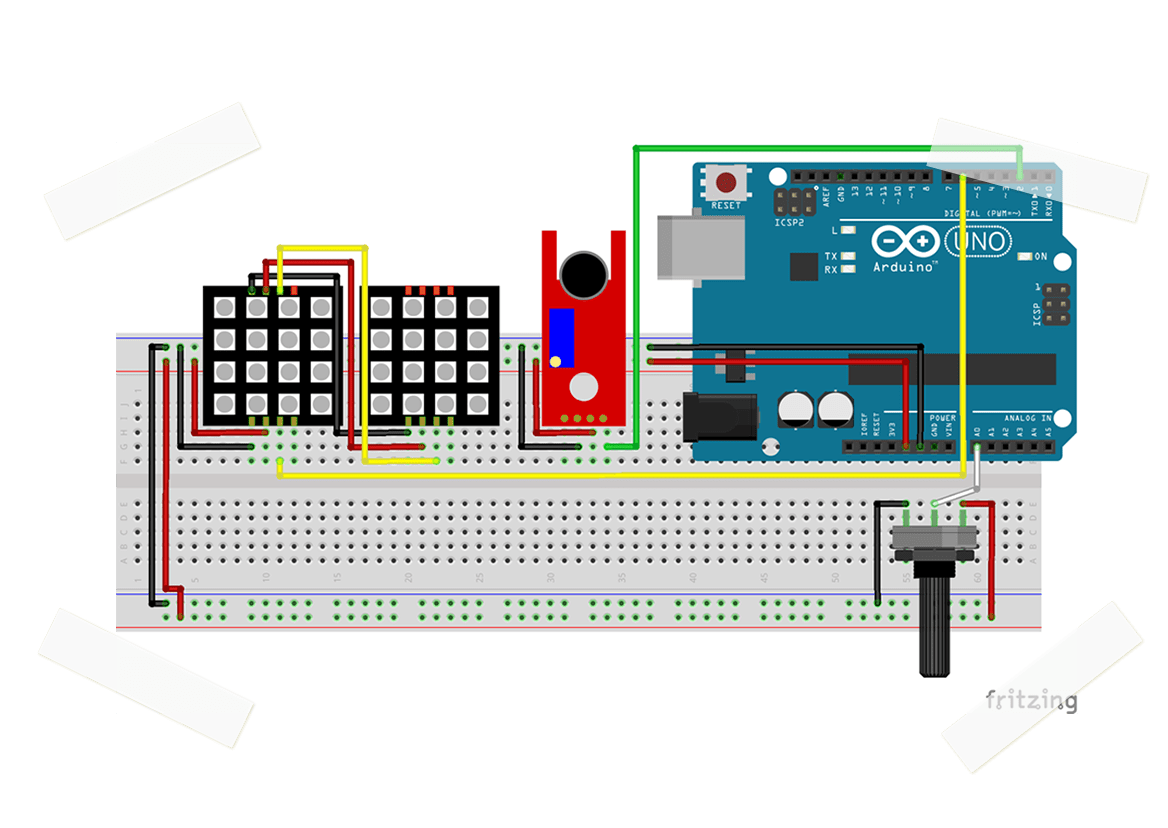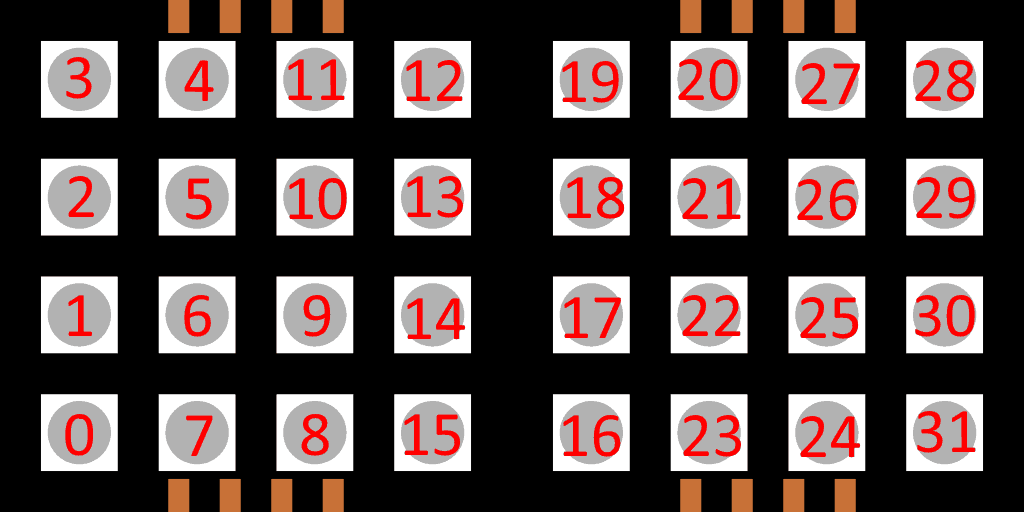# AUDIO VISUALIZER# EXERCISE SOLUTIONS:

### Note: for most of these exercises, there are many different solutions. These are just one possible solution for each question.

Question 1

In loop(), change the line

leds.setPixelColor(0, color);

to

leds.setPixelColor(28, color);

The first argument of the setPixelColor function is the index of the LED. The LEDs are indexed as so:The top right LED’s index is 28, so we change the 0 to a 28.

Question 2

To change the direction of the LED’s, we need to edit the turnOff() function. To change the direction, edit the for loop from:

for(int i = 7; i >= 0 && !complete; i--)

to

for(int i = 0; i <8 && !complete; i++)

Question 3

In the normal program, the color is set with 3 if statements in the for loop at the bottom of the program.
First, we need to remove these if statements.
Next, in the turnOn() function, add:

color = leds.Color(random(255), random(255), random(255));

The random() function returns a random number between 0 and the argument, which is also the range the LED color command can take.

Bonus Question

First, we need to wire the second potentiometer. You can follow the wiring of the first one, but the middle pin needs to be wired to a separate analog input (we will use “A1” for this solution).

Within the setup() function, set the new pin as an analog input:

pinmode(A1, INPUT);

In the loop() function, we need to update the brightness variable: